# The Spacelike-Characteristic Cauchy Problem of General Relativity in Low Regularity

## Abstract

In this paper we study the spacelike-characteristic Cauchy problem for the Einstein vacuum equations. Given initial data on a maximal spacelike hypersurface $$\Sigma \simeq \overline{B_1} \subset {{\mathbb {R}}}^3$$ and the outgoing null hypersurface $${{\mathcal {H}}}$$ emanating from $${\partial }\Sigma$$, we prove a priori estimates for the resulting future development in terms of low-regularity bounds on the initial data at the level of curvature in $$L^2$$. The proof uses the bounded $$L^2$$ curvature theorem , the extension procedure for the constraint equations , Cheeger-Gromov theory in low regularity , the canonical foliation on null hypersurfaces in low regularity  and global elliptic estimates for spacelike maximal hypersurfaces.

This is a preview of subscription content, access via your institution.

## References

1. Adams, R., Fournier, J.: In: Sobolev Spaces. Pure and Applied Mathematics, vol. 140, 2nd edn. Academic Press, Cambridge (2003)

2. An, X., Luk, J.: Trapped surfaces in vaccum arising from mild incoming radiation. Adv. Theo. Math. Phys. 21(1), 1–120 (2017)

3. Bartnik, R.: Existence of maximal surfaces in asymptotically flat spacetimes. Commun. Math. Phys. 94(2), 155–175 (1984)

4. Bruhat, Y.: Théorème d’existence pour certains systèmes d’équations aux dérivées partielles non-linéaires. Acta Math. 88, 141–225 (1952)

5. Bruhat, Y.: Maximal submanifolds and submanifolds with constant mean extrinsic curvature of a Lorentzian manifold. Ann. Scuola Norm. Sup. Pisa Cl. Sci. 4(3), 361–376 (1976)

6. Bruhat, Y., Chruściel, P.T., Martin-Garcia, J.M.: The Cauchy problem on a characteristic cone for the Einstein equations in arbitrary dimensions. Ann. Henri Poincaré 12(3), 419–482 (2011)

7. Christodoulou, D.: The instability of naked singularities in the gravitational collapse of a scalar field. Ann. Math. 149, 183–217 (1999)

8. Christodoulou, D.: The Formation of Black Holes in General Relativity. EMS Monographs in Mathematics, 2009

9. Christodoulou, D., Klainerman, S.: The Global Nonlinear Stability of the Minkowski Space. Princeton Mathematical Series, pp. 10–514. Princeton University Press, Princeton (1993)

10. Chruściel, P.T., Paetz, T.T.: The many ways of the characteristic Cauchy problem. Class. Quantum Gravity 29(14), 145006 (2012)

11. Chruściel, P.T., Paetz, T.T.: Characteristic initial data and smoothness of Scri. I. Framework and results. Ann. Henri Poincaré 16(9), 2131–2162 (2015)

12. Czimek, S.: An extension procedure for the constraint equations. Ann. PDE1 4(1), 122 (2018)

13. Czimek, S.: Boundary harmonic coordinates on manifolds with boundary in low regularity. Commun. Math. Phys. 371(3), 1131–1177 (2019)

14. Czimek, S.: The localised bounded $$L^2$$ curvature theorem. Commun. Math. Phys. 372(1), 71–90 (2019)

15. Czimek, S., Graf, O.: The canonical foliation on null hypersurfaces in low regularity. arXiv, 2019, 69 pp

16. Klainerman, S., Luk, J., Rodnianski, I.: A fully anisotropic mechanism for formation of trapped surfaces in vacuum. Invent. Math. 198(1), 1–26 (2014)

17. Klainerman, S., Rodnianski, I.: Causal geometry of Einstein-vacuum spacetimes with finite curvature flux. Invent. Math. 159(3), 437–529 (2005)

18. Klainerman, S., Rodnianski, I.: A geometric approach to the Littlewood–Paley theory. Geom. Funct. Anal. 16(1), 126–163 (2006)

19. Klainerman, S., Rodnianski, I.: Sharp trace theorems for null hypersurfaces on Einstein metrics with finite curvature flux. Geom. Funct. Anal. 16(1), 164–229 (2006)

20. Klainerman, S., Rodnianski, I.: On the breakdown criterion in general relativity. J. Am. Math. Soc. 23(2), 345–382 (2010)

21. Klainerman, S., Rodnianski, I.: On the formation of trapped surfaces. Acta Math. 208, 211–333 (2012)

22. Klainerman, S., Rodnianski, I., Szeftel, J.: The bounded $$L^2$$ curvature conjecture. Invent. Math. 202(1), 91–216 (2015)

23. Luk, J.: On the local existence for the characteristic initial value problem in general relativity. Int. Math. Res. Not. 20, 4625–4678 (2012)

24. Luk, J., Rodnianski, I.: Local propagation of impulsive gravitational waves. Commun. Pure Appl. Math. 68(4), 511–624 (2015)

25. Luk, J., Rodnianski, I.: Nonlinear interaction of impulsive gravitational waves for the vacuum Einstein equations. Camb. J. Math. 5(4), 435–570 (2017)

26. Penrose, R.: Gravitational Collapse: the Role of General Relativity. Rivista del Nuovo Cimento, Numero Speziale I, 252–276 (1969)

27. Petersen, P.: Convergence Theorems in Riemannian Geometry. Comparison Geometry, pp. 167–202. MSRI Publications, Cambridge (1997)

28. Petersen, P.: Riemannian Geometry. Graduate Texts in Mathematics, 3rd edn., pp. 18–499. Springer, Cham (2016)

29. Rendall, A.: Reduction of the characteristic initial value problem to the Cauchy problem and its applications to the Einstein equations. Proc. R. Soc. Lond. Ser. A 427(1872), 221–239 (1990)

30. Shao, A.: New tensorial estimates in Besov spaces for time-dependent (2+1)-dimensional problems. J. Hyperbolic Differ. Equ. 11(4), 821–908 (2014)

31. Szeftel, J.: Parametrix for wave equations on a rough background I: regularity of the phase at initial time. arXiv:1204.1768, 2012, 145 pp

32. Szeftel, J.: Parametrix for wave equations on a rough background II: construction and control at initial time. arXiv:1204.1769, 2012, 84 pp

33. Szeftel, J.: Parametrix for wave equations on a rough background III: space-time regularity of the phase. Astérisque 401, 321 (2018)

34. Szeftel, J.: Parametrix for wave equations on a rough background IV: control of the error term. arXiv:1204.1771, 2012, 284 pp

35. Szeftel, J.: Sharp Strichartz estimates for the wave equation on a rough background. Annales Scientifiques de l’École Normale Supérieure 49(6), 1279–1309 (2016)

36. Wald, R.: General Relativity. University of Chicago Press, Chicago (1984)

## Acknowledgements

Both authors are very grateful to Jérémie Szeftel for many interesting and stimulating discussions. The second author is supported by the ERC grant ERC-2016 CoG 725589 EPGR.

## Author information

Authors

### Corresponding author

Correspondence to Stefan Czimek.

### Publisher's Note

Springer Nature remains neutral with regard to jurisdictional claims in published maps and institutional affiliations.

## Appendices

### Appendix A. Global Elliptic Estimate for the Second Fundamental Form

In this section we derive a global elliptic estimate for the second fundamental form k of a maximal spacelike hypersurface $$\Sigma \simeq \overline{B_1}$$. We start by deriving an integral identity for Hodge systems on compact Riemannian 3-manifolds with boundary $$\Sigma$$, see Lemma A.2 below. This integral identity is a slight generalisation of Section 8 in  where non-compact manifolds without boundary are considered.

First recall the following notation.

### Definition A.1

Let $$m\ge 0$$ be an integer. For a given totally symmetric $$(m+2)$$-tensor F, define

\begin{aligned} A(F)_{a_1\,\dots a_{m+1}bc} := \nabla _c F_{a_1 \dots a_{m+1} b} - \nabla _b F_{a_1 \dots a_{m+1} c}, \,\, D(F)_{a_1 \dots a_{m+1}} := \nabla ^c F_{a_{1} \dots a_{m+1} c}. \end{aligned}

The following integral identity is a straightforward generalisation of Lemma 4.4.1 in  to manifolds with boundary. The proof is by integration by parts and left to the reader.

### Lemma A.2

(Fundamental integral identity for Hodge systems) Let $$(\Sigma ,g)$$ be a compact Riemannian 3-manifold with boundary and let $$m\ge 0$$ be an integer. Let F be a totally symmetric $$(m+2)$$-tensor on $$\Sigma$$. Then it holds that

\begin{aligned} {\int \limits _\Sigma }\vert \nabla F\,\vert ^2 =&{\int \limits _\Sigma }\frac{1}{2}\vert A(F) \vert ^2 + \vert D(F) \vert ^2 \\&- {\int \limits _\Sigma }\sum \limits _{i=1}^{m+1}\Big ( \mathrm {R}_{\,\,\, a_i b}^{l\,\,\,\,\,\,\,\,\,c} F_{a_1 \dots l \dots a_{m+1}c} +\mathrm {Ric}^{l}_{\,\,\, b} F_{a_1 \dots a_{m+1}l} \Big ) F^{a_1 \dots a_{m+1} b} \\&- {\int \limits _{\partial \Sigma }}F^{a_1 \dots a_{m+1} N} D(F)_{a_1 \dots a_{m+1}} + {\int \limits _{\partial \Sigma }}\nabla _b F_{a_1 \dots a_{m+1} N} F^{a_1 \dots a_{m+1} b}. \end{aligned}

We now use Lemma A.2 to derive global elliptic estimates for the second fundamental form k of a maximal spacelike hypersurface. Let $$({{\mathcal {M}}},\mathbf{g})$$ be a vacuum spacetime and let $$\Sigma \simeq \overline{B_1}$$ be a compact spacelike maximal hypersurface in $${{\mathcal {M}}}$$. By (2.13b), (2.13c) and (2.13d), the second fundamental form k of $$\Sigma$$ satisfies the following Hodge system,

\begin{aligned} \begin{aligned} {{\,\mathrm{div}\,}}_g k =&\,0, \\ {{{\,\mathrm{curl}\,}}}_g\,\, k=&\,H, \\ \mathrm {tr}_g \, k=&\,0. \end{aligned} \end{aligned}

In the notation of Definition A.1, k satisfies

\begin{aligned} A(k)_{iab} = \,\in ^m_{\,\,\,\, ab}H_{im}, \,\, D(k)=0. \end{aligned}
(A.1)

Lemma A.2 together with (A.1) yields the following corollary (see Section 8.3 in  for the case of manifolds without boundary).

### Corollary A.3

(Fundamental global elliptic estimate for k) Let $$({{\mathcal {M}}},\mathbf{g})$$ be a vacuum spacetime and let $$\Sigma \simeq \overline{B_1}$$ be a compact spacelike maximal hypersurface in $${{\mathcal {M}}}$$. Then it holds that

\begin{aligned} \int \limits _{\Sigma } \vert \nabla k \vert ^2 + \frac{1}{4} \vert k \vert ^4-\,\int \limits _{{\partial }\Sigma } \nabla _a k_{bN} k^{ba} \lesssim \int \limits _{\Sigma } \vert {\mathbf {R}} \vert _{{\mathbf {h}}^t}^2, \end{aligned}

where N denotes the outward-pointing unit normal to $${\partial }\Sigma \subset \Sigma$$, T denotes the timelike unit normal to $$\Sigma$$ and $${\mathbf {h}}^t$$ denotes the positive-definite norm on $$\Sigma$$ defined with T, see (2.9).

### Proof

By Lemma A.2 with $$F=k$$, we have

\begin{aligned} \int \limits _{\Sigma } \vert \nabla k \vert ^2 =&\int \limits _{\Sigma } \frac{1}{2}\vert H \vert ^2 -\,\int \limits _{\Sigma } \left( \mathrm {R}^{l\,\,\,\,\,\,\,c}_{\,\,\,ab} k_{lc} + \mathrm {Ric}^l_{\,\,\, b} k_{al}\right) k^{ab} + \int \limits _{{\partial }\Sigma } \nabla _b k_{aN} k^{ab}. \end{aligned}
(A.2)

In dimension $$n=3$$, the full Riemann curvature tensor is determined by $$\mathrm {Ric}$$, yielding

\begin{aligned} \mathrm {R}^l_{\,\,\,abc} k_{lc} k^{ab} = 2 \mathrm {Ric}_{jl} k^{ji} k^{l}_{\,\,\, i} - \frac{1}{2}\mathrm {R}_{scal}\vert k \vert ^2. \end{aligned}

Plugging this into (A.2), we get

\begin{aligned} \int \limits _{\Sigma } \vert \nabla k \vert ^2 =&\int \limits _{\Sigma } \frac{1}{2}\vert H \vert ^2 -\,\int \limits _{\Sigma } \left( 3 \mathrm {Ric}^l_{\,\,\, b} k_{al} k^{ab} - \frac{1}{2}\mathrm {R}_{scal}\vert k\,\vert ^2 \right) + \int \limits _{{\partial }\Sigma } \nabla _b k_{aN} k^{ab}. \end{aligned}
(A.3)

On the one hand, by (2.13f) and (2.13g), we have

\begin{aligned} E_{ij} = \mathrm {Ric}_{ij} - k_{im}k^m_{\,\,j}, \,\, \mathrm {R}_{scal}(g) = \vert k \vert _g^2. \end{aligned}

On the other hand, in dimension $$n=3$$, it holds for symmetric tracefree 2-tensors F that

\begin{aligned} 3\mathrm {tr} (F^4) \ge \vert F \vert ^4. \end{aligned}

Hence it follows from (A.3) that

\begin{aligned} \int \limits _{\Sigma }\frac{1}{2}\vert H \vert ^2 =&\int \limits _{\Sigma } \vert \nabla k \vert ^2 + 3 \mathrm {Ric}_{\,\,a}^{s} k_{bs} k^{ba} - \frac{1}{2}\vert k \vert ^4 -\,\int \limits _{{\partial }\Sigma } \nabla _a k_{bN} k^{ba} \\ =&\int \limits _{\Sigma } \vert \nabla k \vert ^2 + 3 (E_{\,\,a}^{s} + k_m^{\,\, \, s} k^m_{\,\,\, a} ) k_{bs} k^{ba} - \frac{1}{2}\vert k \vert ^4 -\,\int \limits _{{\partial }\Sigma } \nabla _a k_{bN} k^{ba}\\ \ge&\int \limits _{\Sigma } \vert \nabla k \vert ^2 + 3 E_{\,\,a}^{s} k_{bs} k^{ba} + \frac{1}{2}\vert k \vert ^4 -\,\int \limits _{{\partial }\Sigma } \nabla _a k_{bN} k^{ba}. \end{aligned}

Using that $$\vert E \vert ^2 + \vert H \vert ^2 \lesssim \vert {\mathbf {R}}\,\vert _{{\mathbf {h}}^t}^2$$ by (2.8) and (2.11), we obtain

\begin{aligned} \int \limits _{\Sigma } \vert \nabla k \vert ^2 + \frac{1}{4} \vert k \vert ^4-\,\int \limits _{{\partial }\Sigma } \nabla _a k_{bN} k^{ba} \lesssim \int \limits _{\Sigma } \vert {\mathbf {R}} \vert _{{\mathbf {h}}^t}^2. \end{aligned}

This finishes the proof of Corollary A.3. $$\square$$

### Appendix B. Proof of Lemmas 3.5 and 3.6

In this section We Prove Lemmas 3.5 and 3.6.

### 1.1 B.1. Proof of Lemma 3.5

We have to show that on weakly regular balls $$(\Sigma ,g)$$ of radius $$1\le r \le 2$$ with constant $$0<{C_{\mathrm {ball}}}<1/2$$, it holds that

\begin{aligned} \begin{aligned} \Vert F \Vert _{L^2({\partial }\Sigma )} \lesssim&\, \Vert F \Vert _{L^2(\Sigma )} + \Vert \nabla F \Vert _{L^2(\Sigma )}, \\ \Vert F \Vert _{L^4({\partial }\Sigma )} \lesssim&\,\Vert F \Vert _{L^2(\Sigma )} + \Vert \nabla F \Vert _{L^2(\Sigma )}, \end{aligned} \end{aligned}
(B.1)

and

\begin{aligned} \Vert F \Vert _{H^{1/2}({\partial }\Sigma )} \lesssim&\, \Vert F \Vert _{L^2(\Sigma )} + \Vert \nabla F \Vert _{L^2(\Sigma )}. \end{aligned}
(B.2)

First, the estimates (B.1) are straightforward, see, for example, Lemma 3.26 and Corollary 3.27 in  for a concise proof.

We turn to the proof of (B.2). On the one hand, by Sections 7.50 to 7.56 in , for each coordinate patch $$U \subset {\partial }\overline{B_r}$$ and smooth open set $$V \subset \overline{B_r}$$ with $$U \subset \left( V \cap {\partial }\overline{B_r}\right)$$, it holds that

\begin{aligned} H^1(V) \hookrightarrow H^{1/2}(U), \end{aligned}

where $$H^{1/2}(U)$$ denotes a local coordinate-defined fractional Sobolev space on U.

On the other hand, if $$g_{ij} \in H^2({B_r})$$ then in particular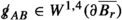in local coordinates. By Proposition 3.2 in , this control suffices to compare the coordinate-defined spaces $$H^{1/2}(U)$$ with the space $$H^{1/2}({\partial }\overline{B_r})$$ defined in Definition 3.2, see also Appendix B of . This finishes the proof of (B.2).This finishes the proof of Lemma 3.5.

### 1.2 B.2. Proof of Lemma 3.6

Let $$1\le r_0 \le 2$$ and $$0<{C_{\mathrm {ball}}}<1/2$$ be two real numbers. Let $$(\Sigma ,g)$$ be a weakly regular ball of radius $$r_0$$ with constant $${C_{\mathrm {ball}}}$$. Let F be a tensor on $$\Sigma$$. We have to show that

\begin{aligned} \Vert F \Vert _{L^2(\Sigma )} \lesssim \Vert \nabla F \Vert _{L^2(\Sigma )} + \Vert F \Vert _{L^2({\partial }\Sigma )}. \end{aligned}

Define spherical coordinates $$(r,\theta ^1, \theta ^2)$$ with $$r \in [0,r_0]$$ and $$(\theta ^1,\theta ^2)\in {{\mathbb {S}}}^2$$ on $$\Sigma \simeq \overline{B_{r_0}}$$ as in Section 2.4. Let $${\gamma }$$ and $$d \mu _{\gamma }$$ on $${{\mathcal {S}}}_r$$ denote the standard round metric of radius $$r>0$$ and its volume element, respectively. Denote by $${\overset{\circ }{\gamma }}$$ and $$d \mu _{{\overset{\circ }{\gamma }}}$$ the standard round metric on the unit sphere and its volume element, respectively. Note that $$\gamma = r^2 {\overset{\circ }{\gamma }}$$. By the fundamental theorem of calculus and using that $${\partial }\Sigma = {{\mathcal {S}}}_{r_0}$$, we have for $$0<r \le r_0$$,

\begin{aligned} \int \limits _{{{\mathcal {S}}}_r} \vert F \vert _g^2 \, d\mu _{\overset{\circ }{\gamma }}=&\int \limits _{r_0}^{r} {\partial }_{r'} \left( \, \int \limits _{{{\mathcal {S}}}_{r'}} \vert F \vert ^2_g d\mu _{\overset{\circ }{\gamma }}\right) dr' + \int \limits _{{\partial }\Sigma } \vert F \vert _g^2 d\mu _{\overset{\circ }{\gamma }}\\ =&\int \limits _{r_0}^r \left( \, \int \limits _{{{\mathcal {S}}}_{r'}} \nabla _{{\partial }_{r'}} F \cdot F \, d\mu _{\overset{\circ }{\gamma }}\right) dr' + \int \limits _{{\partial }\Sigma } \vert F \vert _g^2 d\mu _{\overset{\circ }{\gamma }}\\ \le&\left\| \vert {\partial }_r \vert _g^2 \right\| _{L^\infty (\Sigma )} \left( \int \limits _{r_0}^r \int \limits _{{{\mathcal {S}}}_{r'}} \vert \nabla F \vert ^2_g d\mu _{\overset{\circ }{\gamma }}dr' \right) ^{1/2} \left( \int \limits _{r_0}^r \int \limits _{{{\mathcal {S}}}_{r'}} \vert F \vert ^2_g d\mu _{\overset{\circ }{\gamma }}dr' \right) ^{1/2}\\&+ \int \limits _{{\partial }\Sigma } \vert F \vert _g^2 d\mu _{\overset{\circ }{\gamma }}. \end{aligned}

Using that by definition of the spherical coordinates on $$B_{r_0}$$ and the weakly regular ball property of $$(\Sigma ,g)$$,

\begin{aligned} \vert {\partial }_r \vert _g^2 = g_{rr} = \frac{x^i}{r} \frac{x^j}{r} g_{ij} \le (1+ {C_{\mathrm {ball}}})\frac{x^i}{r} \frac{x^j}{r} e_{ij} =1+{C_{\mathrm {ball}}}, \end{aligned}

we can estimate the right-hand side as follows,

\begin{aligned} \int \limits _{{{\mathcal {S}}}_r} \vert F \vert _g^2 \, d\mu _{\overset{\circ }{\gamma }}\lesssim&\left( \int \limits _{r_0}^r \int \limits _{{{\mathcal {S}}}_{r'}} \vert \nabla F \vert ^2_g d\mu _{\overset{\circ }{\gamma }}dr' \right) ^{1/2} \left( \int \limits _{r_0}^r \int \limits _{{{\mathcal {S}}}_{r'}} \vert F \vert ^2_g d\mu _{\overset{\circ }{\gamma }}dr' \right) ^{1/2}+ \int \limits _{{\partial }\Sigma } \vert F \vert _g^2 d\mu _{\overset{\circ }{\gamma }}\\ \lesssim&\,\frac{1}{r^2} \left( \int \limits _{r_0}^r \int \limits _{{{\mathcal {S}}}_{r'}} \vert \nabla F \vert ^2_g d\mu _{\gamma } dr' \right) ^{1/2} \left( \int \limits _{r_0}^r \int \limits _{{{\mathcal {S}}}_{r'}} \vert F \vert ^2_g d\mu _{\gamma } dr' \right) ^{1/2}+ \int \limits _{{\partial }\Sigma } \vert F \vert _g^2 d\mu _{\overset{\circ }{\gamma }}\\ \lesssim&\, \frac{1}{r^2} \left( \, \int \limits _{B_{r_0}} \vert \nabla F \vert ^2_g d\mu _{e} \right) ^{1/2} \left( \, \int \limits _{B_{r_0}} \vert F \vert ^2_g d\mu _{e} \right) ^{1/2} + \int \limits _{{\partial }\Sigma } \vert F \vert _g^2 d\mu _{\overset{\circ }{\gamma }}\\ \lesssim&\,\frac{1}{r^2} \Vert \nabla F \Vert _{L^2(B_{r_0})}\Vert F \Vert _{L^2(B_{r_0})} + \int \limits _{{\partial }\Sigma } \vert F \vert _g^2 d\mu _{\overset{\circ }{\gamma }}, \end{aligned}

where $$d\mu _e$$ denotes the measure with respect to the standard Euclidean metric e in Cartesian coordinates, and we used in the last inequality that $$\Sigma$$ is a weakly regular ball to compare the Euclidean integral with the g-dependent norm.

Multiplying the above by $$r^2$$ and using that by Lemmas 3.1, 3.5 and 3.7,

\begin{aligned} \int \limits _{{\partial }\Sigma } \vert F \vert _g^2 d\mu _{r_0^2{\overset{\circ }{\gamma }}} \lesssim \Vert F \Vert _{L^2({\partial }\Sigma )}^2, \end{aligned}

we get that for $$0<r\le r_0$$,

\begin{aligned} \begin{aligned} \int \limits _{{{\mathcal {S}}}_r} \vert F \vert _g^2 \, d\mu _\gamma \lesssim&\,\Vert \nabla F \Vert _{L^2(\Sigma )} \Vert F \Vert _{L^2(\Sigma )} + \frac{r^2}{r_0^2} \int \limits _{{\partial }\Sigma } \vert F \vert _g^2 d\mu _{r_0^2{\overset{\circ }{\gamma }}} \\ \lesssim&\, \Vert \nabla F \Vert _{L^2(\Sigma )} \Vert F \Vert _{L^2(\Sigma )} + \frac{r^2}{r_0^2} \Vert F\Vert ^2_{L^2({\partial }\Sigma )}. \end{aligned} \end{aligned}
(B.3)

By (B.3) and using that $$(\Sigma ,g)$$ is a weakly regular ball of radius $$r_0$$, we get that

\begin{aligned} \Vert F \Vert _{L^2(\Sigma )}^2&\lesssim \int \limits _{B_{r_0}} \vert F \vert ^2_g d\mu _e \lesssim \int \limits _{0}^{r_0} \left( \, \int \limits _{{{\mathcal {S}}}_r} \vert F \vert _g^2 \, d\mu _\gamma \right) dr \\&\lesssim \Vert \nabla F \Vert _{L^2(\Sigma )} \Vert F \Vert _{L^2(\Sigma )} + \Vert F\Vert ^2_{L^2({\partial }\Sigma )}, \end{aligned}

which implies that

\begin{aligned} \Vert F \Vert _{L^2(\Sigma )} \lesssim \Vert \nabla F \Vert _{L^2(\Sigma )} + \Vert F \Vert _{L^2({\partial }\Sigma )}. \end{aligned}

This finishes the proof of Lemma 3.6.

### Appendix C. Proof of Lemma 5.4

In this section we prove Lemma 5.4. Let (Mg) be a smooth compact Riemannian 3-manifold with boundary such that $$M \simeq \overline{B_1} \subset {{\mathbb {R}}}^3$$ and for a real number $$1\le t \le 2$$,

\begin{aligned} \mathrm {Ric}= 0 \text { on } M, \,\, \mathrm {tr}\, \Theta =\frac{2}{t}, \, {\widehat{\Theta }}=0 \text { on } {\partial }M. \end{aligned}
(C.1)

We have to prove that

\begin{aligned} (M,g) \cong (\overline{B_t},e). \end{aligned}

First, by the Gauss equation

\begin{aligned} 2K = \left( \mathrm {tr}\Theta \right) ^2- \vert \Theta \vert ^2 + R_{\mathrm {scal}} - 2 \mathrm {Ric}(N,N), \end{aligned}

it follows that the Gauss curvature of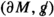is constant $$K=\frac{1}{t^2}$$. Hence by classical differential geometry, there exist coordinates $$(\theta ^1, {\theta }^2)$$ on $${\partial }M$$ such that(C.2)

Second, by the smoothness of (Mg), there exists in an open neighbourhood $${{\mathcal {U}}}\subset M$$ of $${\partial }M \subset M$$ a so-called Gaussian coordinate system $$({r},{\theta }^1,{\theta }^2)$$, see for example Section 3.3 in , which coincide with (C.2) on $${\partial }M$$ and are such that for some small real number $$\delta >0$$,

\begin{aligned}&{{\mathcal {U}}}= \{ {r}\in (t-\delta ,t]\}, \\&{{\mathcal {U}}}\cap {\partial }M = \{ {r}=t\}, \\&\nabla _{{\partial }_r}{\partial }_r =0, \\&{\partial }_r \vert _{{\partial }M} \text { is normal to } {\partial }M, \\&g({\partial }_r,{\partial }_r)=1. \end{aligned}

In such coordinates we can express g aswhere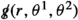denotes the induced metric on the level sets $$S_r$$ of r. In particular, by (C.2) it holds that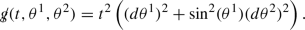Third, we claim that the Riemannian manifold (Mg) smoothly extends onto $${{\mathbb {R}}}^3 \setminus \overline{B_t}$$ when identifying $${\partial }M = {\partial }\overline{B_t}\subset {{\mathbb {R}}}^3$$. It suffices to show that the induced metric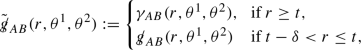is smooth across $$\{r=t\}$$. Here $$\gamma _{AB}(r,\theta ^1,\theta ^2)$$ is the standard round metric of radius r. In the following calculations we denoteon $${{\mathcal {U}}}$$ simply by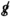.

By (C.2) it follows thatis continuous across $$\{r=t\}$$. Further, on the one hand, on $${\partial }\overline{B_t}\subset {{\mathbb {R}}}^3$$, it holds that

\begin{aligned} {\partial }_r \gamma _{AB}\vert _{r=t} =&2 t {\overset{\circ }{\gamma }}_{AB}, \,\, {\partial }_r^2 \gamma _{AB}\vert _{r=t} = 2 {\overset{\circ }{\gamma }}_{AB}, \,\, {\partial }_r^{m} \gamma _{AB}\vert _{r=t}=0 \text { for } m\ge 3, \end{aligned}
(C.3)

where $${\overset{\circ }{\gamma }}_{AB}$$ denotes the metric components of the standard round metric on $${\mathbb {S}}^2$$.

On the other hand, by construction of $$(r,\theta ^1, \theta ^2)$$ it holds on $${{\mathcal {U}}}$$ that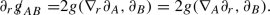(C.4)

By (C.1), (C.2) and (C.4), on $${\partial }M$$ we thus have that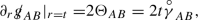(C.5)

Differentiating (C.4) in r yields(C.6)

where we used that $$\mathrm {Rm}=0$$ in (Mg) and $$\nabla _{{\partial }_r}{\partial }_r=0$$ in Gaussian coordinates in $${{\mathcal {U}}}$$. From (C.5) and (C.6) and using that $$a=1$$, it follows that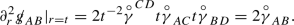(C.7)

Differentiating (C.4) further in r shows that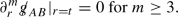(C.8)

Comparing (C.3) with (C.5), (C.7) and (C.8) shows that all r-derivatives ofagree on $$r=t$$. Hence (Mg) smoothly extends as Riemannian manifold onto $$({{\mathbb {R}}}^3 \setminus \overline{B_t},e)$$.

The resulting smooth Riemannian 3-manifold is in particular flat, complete and has cubic volume growth of geodesic balls. By Proposition 4.4 in  it must therefore be isometric to $$({{\mathbb {R}}}^3,e)$$. We conclude that by the above construction,

\begin{aligned} (M,g) \cong ({{\mathbb {R}}}^3 \setminus \left( {{\mathbb {R}}}^3 \setminus \overline{B_t}\right) ,e) \cong (\overline{B_t},e), \end{aligned}

which finishes the proof of Lemma 5.4.

### Appendix D. Comparison Estimates Between Maximal Spacelike Foliations

In this section we prove Lemma 4.6. By assumption, for real numbers $$D>0$$ and $$\varepsilon >0$$, $${{\mathcal {M}}}_{t^*}$$ is foliated by maximal spacelike hypersurface $$(\Sigma _t)_{1\le t\,\le t^*}$$ given as level sets of a time function t with $$\Sigma _1 = \Sigma$$ and satisfying

\begin{aligned} \begin{aligned}&\Vert \mathbf{R}\Vert _{L^\infty _{t} L^2({\Sigma }_{t})} + \Vert \mathrm {Ric}\Vert _{L^\infty _tL^2(\Sigma _t)} \le \,D\varepsilon , \\&\Vert \nabla k \Vert _{L^\infty _{ t} L^2({\Sigma }_{ t})} + \Vert {k} \Vert _{L^\infty _{ t} L^2({\Sigma }_{ t})} \le \, D\varepsilon , \\&\Vert n-1 \Vert _{L^\infty ({{\mathcal {M}}}_{t^*})} + \Vert \nabla n \Vert _{L^\infty _tL^2(\Sigma _t)} +\Vert \nabla ^2 n \Vert _{L^\infty _tL^2(\Sigma _t)}\lesssim \,D\varepsilon . \end{aligned} \end{aligned}
(D.1)

Let T denote the timelike unit normal to $$\Sigma _t$$.

Moreover, by assumption there is another foliation on $${{\mathcal {M}}}_{t^*}$$ (constructed by the bounded $$L^2$$ curvature theorem) of maximal hypersurfaces $$(\widetilde{\Sigma }_{{{\tilde{t}}}})_{0 \le {{\tilde{t}}}\,\le t^*}$$ given as level sets of a time function $${{\tilde{t}}}$$ with $$\Sigma _{t^*} = \widetilde{\Sigma }_{t^*}$$ and satisfying

\begin{aligned} \begin{aligned}&\Vert \mathbf{R}\Vert _{L^\infty _{{{\tilde{t}}}} L^2(\widetilde{\Sigma }_{{{\tilde{t}}}})} + \Vert \widetilde{\mathrm {Ric}}\Vert _{L^\infty _{{{\tilde{t}}}} L^2(\widetilde{\Sigma }_{{{\tilde{t}}}})} \lesssim \,{C_{\mathrm {ball}}}, \\&\Vert {{\widetilde{\nabla }}}{\tilde{k}} \Vert _{L^\infty _{{{\tilde{t}}}} L^2(\widetilde{\Sigma }_{{{\tilde{t}}}})} + \Vert {\tilde{k}} \Vert _{L^\infty _{{{\tilde{t}}}} L^2(\widetilde{\Sigma }_{{{\tilde{t}}}})}+ \Vert \mathbf{D}\widetilde{\pi } \Vert _{L^\infty _{{\tilde{t}}}L^2({{\widetilde{\Sigma }}}_{{\tilde{t}}})} \lesssim \,{C_{\mathrm {ball}}}, \\&\Vert {\tilde{n}}-1 \Vert _{L^\infty ({{\mathcal {M}}}_{t^*})} + \Vert {{\widetilde{\nabla }}}{\tilde{n}} \Vert _{L^\infty ({{\mathcal {M}}}_{t^*})} + \Vert {{\widetilde{\nabla }}}^2 {\tilde{n}} \Vert _{L^\infty _{{\tilde{t}}}L^2(\widetilde{\Sigma }_{{\tilde{t}}})} \lesssim \, {C_{\mathrm {ball}}}, \end{aligned} \end{aligned}
(D.2)

where $$\widetilde{\mathrm {Ric}}$$, $${{\widetilde{\nabla }}}$$ and $${\tilde{k}}$$ denote the Ricci curvature, covariant derivative and the second fundamental form on $$\widetilde{\Sigma }_{{{\tilde{t}}}}$$, respectively. Let $${{\widetilde{T}}}$$ denote the timelike unit normal to $$\widetilde{\Sigma }_{{{\tilde{t}}}}$$.

We need to show that for $$\varepsilon >0$$ and $${C_{\mathrm {ball}}}>0$$ sufficiently small, for $$1\le t \le t^*$$,

\begin{aligned} \left\| {{\tilde{\nu }}}-1\right\| _{L^\infty _t L^\infty (\Sigma _t)} \lesssim&\,{C_{\mathrm {ball}}}, \end{aligned}
(D.3)
\begin{aligned} \Vert \widetilde{k} \Vert _{L^\infty _t L^4(\Sigma _t)}\,\lesssim&\, {C_{\mathrm {ball}}}, \end{aligned}
(D.4)

where the angle $${\tilde{\nu }}$$ between T and $${{\widetilde{T}}}$$ is defined as

\begin{aligned} {{\tilde{\nu }}}:= -\mathbf{g}(T, {{\widetilde{T}}}). \end{aligned}
(D.5)

The proof of (D.3) and (D.4) is based on a standard continuity argument going backwards in t and starting at $$\Sigma _{t^*}={{\widetilde{\Sigma }}}_{t^*}$$ where by construction

\begin{aligned} {{\tilde{\nu }}}=1, \,\, k={{\tilde{k}}}. \end{aligned}

In the following, we only discuss the bootstrap assumption and its improvement.

Bootstrap assumption. Let $$1\le t_0^*<t^*$$ be a real. Assume that for a large constant $$M>0$$, for $$t_0^*\le t \le t^*$$,

\begin{aligned} \begin{aligned} \left\| {{\tilde{\nu }}}-1\right\| _{L^\infty _tL^\infty (\Sigma _t)} \le M {C_{\mathrm {ball}}}. \end{aligned}\end{aligned}
(D.6)

First consequences of the bootstrap assumption. Let $$({{\tilde{e}}}_i)_{i=1,2,3}$$ be an orthonormal frame on $$\widetilde{\Sigma }_{{\tilde{t}}}$$ and let $${{\tilde{e}}}_0= {{\widetilde{T}}}$$. Expressing T as

\begin{aligned} T={{\tilde{\nu }}}{{\widetilde{T}}}+ \mathbf{g}(T, {\tilde{e}}_i) {\tilde{e}}_i, \end{aligned}

and using that $$\mathbf{g}(T,T)=-1$$, it follows that

\begin{aligned} -1 = - \vert {{\tilde{\nu }}}\vert ^2 + \sum \limits _{i=1,2,3} \vert \mathbf{g}(T,{{\tilde{e}}}_i)\vert ^2. \end{aligned}

This implies by the bootstrap assumption (D.6) for $${C_{\mathrm {ball}}}>0$$ sufficiently small that for $$t_0^*\le t \le t^*$$,

\begin{aligned} \, \left\| \mathbf{g}(T,{{\tilde{e}}}_i)\right\| _{L^\infty _tL^\infty (\Sigma _t)} \lesssim \sqrt{M{C_{\mathrm {ball}}}}. \end{aligned}
(D.7)

Let $$(e_i)_{i=1,2,3}$$ be the orthonormal frame tangent to $$\Sigma _t$$ constructed by the Gram-Schmidt method applied to the $$\Sigma _t$$-tangential frame

\begin{aligned} ({{\tilde{e}}}_1+\mathbf{g}({{\tilde{e}}}_1,T)T,{{\tilde{e}}}_2+\mathbf{g}({{\tilde{e}}}_2,T)T,{{\tilde{e}}}_3+\mathbf{g}({{\tilde{e}}}_3,T)T), \end{aligned}

and set moreover $$e_0 := T$$. By the Gram-Schmidt construction and (D.6) and (D.7), we get that for $${C_{\mathrm {ball}}}>0$$ sufficiently small, for $$t_0^*\le t \le t^*$$,

\begin{aligned} \begin{aligned}&\sum \limits _{i=1}^3 \left( \left\| \mathbf{g}(e_i,{{\tilde{e}}}_i)-1\right\| _{L^\infty _tL^\infty (\Sigma _t)} +\sum \limits _{j\ne i} \left\| \mathbf{g}({{\tilde{e}}}_i,e_j)\right\| _{L^\infty _tL^\infty (\Sigma _t)} + \left\| \mathbf{g}({{\widetilde{T}}},e_i)\right\| _{L^\infty _tL^\infty (\Sigma _t)} \right) \\&\quad \lesssim \sqrt{M{C_{\mathrm {ball}}}}. \end{aligned} \end{aligned}
(D.8)

Improvement of the bootstrap assumption. First, by definition of $${{\tilde{\nu }}}$$, see (D.5), and using that $$\mathbf{D}_{T}T = n^{-1}\nabla n$$,

\begin{aligned} \begin{aligned} T ({{\tilde{\nu }}}) =&-\mathbf{g}(\mathbf{D}_{T} T,{{\widetilde{T}}}) -\mathbf{g}(T,\mathbf{D}_{T}{{\widetilde{T}}}) \\ =&- \left( \mathbf{g}({{\widetilde{T}}},e_i)\mathbf{g}(\mathbf{D}_{T}T,e_i) + \mathbf{g}(T,{{\tilde{e}}}_i) \mathbf{g}({{\tilde{e}}}_i, \mathbf{D}_{\mathbf{g}(T,{{\tilde{e}}}_j){{\tilde{e}}}_j-\mathbf{g}(T,{{\tilde{e}}}_0){{\tilde{e}}}_0} {{\widetilde{T}}}) \right) \\ =&-\left( \mathbf{g}({{\widetilde{T}}},e_i)(n^{-1}\nabla _{e_i} n) - \mathbf{g}(T,{{\tilde{e}}}_j) \mathbf{g}(T,{{\tilde{e}}}_i) \widetilde{k}_{ij} + {{\tilde{\nu }}}\mathbf{g}(T,{{\tilde{e}}}_i) (\widetilde{n}^{-1}\widetilde{\nabla }_{{{\tilde{e}}}_i}\widetilde{n}) \right) . \end{aligned} \end{aligned}
(D.9)

Define shift-free coordinates $$(t,x^1,x^2,x^3)$$ on $${{\mathcal {M}}}_{t^*}$$ by transporting $$(x^1,x^2,x^3)$$ from $$\Sigma _{t^*}$$ backwards along T. Then it holds that $${\partial }_t = n^{-1} T$$, and we get from (D.9) that

\begin{aligned} {\partial }_t {{\tilde{\nu }}}= -n^{-1}\left( \mathbf{g}({{\widetilde{T}}},e_i)(n^{-1}\nabla _{e_i} n) - \mathbf{g}(T,{{\tilde{e}}}_j) \mathbf{g}(T,{{\tilde{e}}}_i) \widetilde{k}_{ij} + {{\tilde{\nu }}}\mathbf{g}(T,{{\tilde{e}}}_i) (\widetilde{n}^{-1}\widetilde{\nabla }_{{{\tilde{e}}}_i}\widetilde{n}) \right) . \end{aligned}

Integrating this equation in t, using that $${{\tilde{\nu }}}\vert _{t=t^*}=1$$ and applying (D.1), (D.2), (D.6), (D.7), (D.8) and Lemma 3.4, we get that for $$\varepsilon >0$$ and $${C_{\mathrm {ball}}}>0$$ sufficiently small, for $$t^*_0 \le t \le t^*$$, it holds that

\begin{aligned} \begin{aligned} \left\| {{\tilde{\nu }}}-1\right\| _{L^\infty _t L^4(\Sigma _t)} \lesssim&\, \Vert {\mathbf{g}({{\widetilde{T}}},e_i)}\Vert _{L^\infty ({{\mathcal {M}}}_{[t_0^*,t^*]})}\Vert {\nabla _{e_i} n}\Vert _{L^4({{\mathcal {M}}}_{[t_0^*,t^*]})} \\&+ \Vert {\mathbf{g}(T,{{\tilde{e}}}_i)}\Vert _{L^\infty ({{\mathcal {M}}}_{[t_0^*,t^*]})} \Vert {\mathbf{g}(T,{{\tilde{e}}}_j)}\Vert _{L^\infty ({{\mathcal {M}}}_{[t_0^*,t^*]})}\Vert {\widetilde{k}_{ij}}\Vert _{L^4({{\mathcal {M}}}_{[t_0^*,t^*]})} \\&+ \Vert {{{\tilde{\nu }}}}\Vert _{L^\infty ({{\mathcal {M}}}_{[t_0^*,t^*]})}\Vert {\mathbf{g}(T,{{\tilde{e}}}_i)}\Vert _{L^\infty ({{\mathcal {M}}}_{[t_0^*,t^*]})}\Vert {\widetilde{\nabla }_{{{\tilde{e}}}_i}\widetilde{n}}\Vert _{L^4({{\mathcal {M}}}_{[t_0^*,t^*]})} \\ \lesssim&\, \Vert {\mathbf{g}({{\widetilde{T}}},e_i)}\Vert _{L^\infty ({{\mathcal {M}}}_{[t_0^*,t^*]})}\\&\left( \Vert {\nabla n}\Vert _{L^\infty _tL^2(\Sigma _t)}+\Vert {\nabla ^2 n}\Vert _{L^\infty _tL^2(\Sigma _t)} \right) \\&+ \Vert {\mathbf{g}(T,{{\tilde{e}}}_i)}\Vert _{L^\infty ({{\mathcal {M}}}_{[t_0^*,t^*]})} \Vert {\mathbf{g}(T,{{\tilde{e}}}_j)}\Vert _{L^\infty ({{\mathcal {M}}}_{[t_0^*,t^*]})}\\&\left( \Vert {\widetilde{k}}\Vert _{L^\infty _{{\tilde{t}}}L^2(\widetilde{\Sigma }_{{\tilde{t}}})}+\Vert {\widetilde{\nabla } \widetilde{k}}\Vert _{L^\infty _{{\tilde{t}}}L^2(\widetilde{\Sigma }_{{\tilde{t}}})} \right) \\&+ \Vert {{{\tilde{\nu }}}}\Vert _{L^\infty ({{\mathcal {M}}}_{[t_0^*,t^*]})}\Vert {\mathbf{g}(T,{{\tilde{e}}}_i)}\Vert _{L^\infty ({{\mathcal {M}}}_{[t_0^*,t^*]})}\\&\left( \Vert {\widetilde{\nabla }\widetilde{n}}\Vert _{L^\infty _{{\tilde{t}}}L^2(\widetilde{\Sigma }_{{\tilde{t}}})}+\Vert {\widetilde{\nabla }^2 \widetilde{n}}\Vert _{L^\infty _{{\tilde{t}}}L^2(\widetilde{\Sigma }_{{\tilde{t}}})} \right) \\ \lesssim&\, \sqrt{M{C_{\mathrm {ball}}}} (D\varepsilon ) + \sqrt{M{C_{\mathrm {ball}}}} {C_{\mathrm {ball}}}, \end{aligned} \end{aligned}
(D.10)

where $${{\mathcal {M}}}_{[t_0^*,t^*]}$$ denotes the spacetime region $$t_0^*\le t \le t^*$$.

Second, by definition of $${{\tilde{\nu }}}$$ in (D.5), for $$i=1,2,3$$,

\begin{aligned} \nabla _{e_i}{{\tilde{\nu }}}=&-\mathbf{g}(\mathbf{D}_{e_i}T,{{\widetilde{T}}}) - \mathbf{g}(T,\mathbf{D}_{e_i}{{\widetilde{T}}}) \\ =&- \left( \mathbf{g}({{\widetilde{T}}},e_j)k_{ij} + \mathbf{g}(T,{{\tilde{e}}}_j) \mathbf{g}({{\tilde{e}}}_j, \mathbf{D}_{\mathbf{g}(e_i,{{\tilde{e}}}_l){{\tilde{e}}}_l-\mathbf{g}(e_i,{{\tilde{e}}}_0){{\tilde{e}}}_0} {{\widetilde{T}}}) \right) \\ =&- \left( \mathbf{g}({{\widetilde{T}}},e_j)k_{ij} - \mathbf{g}(T,{{\tilde{e}}}_j) \mathbf{g}(e_i,{{\tilde{e}}}_l) \widetilde{k}_{lj}+ \mathbf{g}(T,{{\tilde{e}}}_j) \mathbf{g}(e_i,{{\widetilde{T}}}) (\widetilde{n}^{-1} \widetilde{\nabla }_{{{\tilde{e}}}_j} \widetilde{n}) \right) \end{aligned}

By (D.1), (D.2), (D.8) and Lemma 3.4, we have for $${C_{\mathrm {ball}}}>0$$ and $$\varepsilon >0$$ sufficiently small,

\begin{aligned} \begin{aligned} \left\| \nabla _{e_i} {{\tilde{\nu }}}\right\| _{L^\infty _t L^4(\Sigma _t)} \lesssim&\, \Vert {\mathbf{g}({{\widetilde{T}}},e_j)}\Vert _{L^\infty ({{\mathcal {M}}}_{[t_0^*,t^*]})}\Vert {k_{ij}}\Vert _{L^\infty _t L^4(\Sigma _t)} \\&+ \Vert {\mathbf{g}(T,{{\tilde{e}}}_j)}\Vert _{L^\infty ({{\mathcal {M}}}_{[t_0^*,t^*]})}\Vert {\mathbf{g}(e_i,{{\tilde{e}}}_l)}\Vert _{L^\infty ({{\mathcal {M}}}_{[t_0^*,t^*]})}\Vert {\widetilde{k}_{lj}}\Vert _{L^\infty _t L^4(\Sigma _t)} \\&+ \Vert {\mathbf{g}(T,{{\tilde{e}}}_j)}\Vert _{L^\infty ({{\mathcal {M}}}_{[t_0^*,t^*]})}\Vert {\mathbf{g}(e_i,{{\widetilde{T}}})}\Vert _{L^\infty ({{\mathcal {M}}}_{[t_0^*,t^*]})}\Vert {\widetilde{\nabla }_{{{\tilde{e}}}_j} \widetilde{n}}\Vert _{L^\infty _t L^\infty (\Sigma _t)} \\ \lesssim&\sqrt{M{C_{\mathrm {ball}}}} D\varepsilon + \sqrt{M{C_{\mathrm {ball}}}}\Vert { \widetilde{k}_{lj}}\Vert _{L^\infty _t L^4(\Sigma _t)} +(M{C_{\mathrm {ball}}}) {C_{\mathrm {ball}}}. \end{aligned} \end{aligned}
(D.11)

By (D.10), (D.11) and Lemma 3.4, it follows that

\begin{aligned} \left\| {{\tilde{\nu }}}-1\right\| _{L^\infty ({{\mathcal {M}}}_{[t_0^*,t^*]})} \lesssim \sqrt{M{C_{\mathrm {ball}}}} (D\varepsilon +\sqrt{M{C_{\mathrm {ball}}}}{C_{\mathrm {ball}}}) + \sqrt{M{C_{\mathrm {ball}}}} \Vert {\widetilde{k}_{lj} }\Vert _{L^\infty _t L^4(\Sigma _t)}. \end{aligned}
(D.12)

To estimate the remaining term $$\Vert {\widetilde{k}_{lj} }\Vert _{L^\infty _t L^4(\Sigma _t)}$$ on the right-hand side of (D.12), we apply the following technical lemma whose proof is postponed to the end of this section.

### Lemma 5.5

(Technical lemma) Under the assumption of (D.1), (D.2), (D.6) for $${C_{\mathrm {ball}}}>0$$ and $$\varepsilon >0$$ sufficiently small, it holds for every scalar function f on $${{\mathcal {M}}}_{t^*}$$ that for $$t_0^*\le t \le t^*$$,

\begin{aligned} \left\| f\right\| _{L^\infty _t L^4(\Sigma _t)} \lesssim&\left\| \mathbf{D}f \right\| _{L^\infty _{{\tilde{t}}}L^2({{\widetilde{\Sigma }}}_{{\tilde{t}}})}^{1/4} \left\| f\right\| _{L^\infty _{{\tilde{t}}}L^6({{\widetilde{\Sigma }}}_{{\tilde{t}}})}^{3/4} + \left\| f\right\| _{L^4(\Sigma _{t^*})}. \end{aligned}

Applying Lemma 5.5 to $$f = \widetilde{k}_{ij}$$, we have for $$\varepsilon >0$$ and $${C_{\mathrm {ball}}}>0$$ sufficiently small, for $$t_0^*\le t \le t^*$$,

\begin{aligned} \begin{aligned} \Vert \widetilde{k}_{lj}\Vert _{L^\infty _t L^4(\Sigma _t)} \lesssim&\, \Vert \mathbf{D}(\widetilde{k}_{lj})\Vert _{L^\infty _{{\tilde{t}}}L^2({{\widetilde{\Sigma }}}_{{\tilde{t}}})}^{1/4} \Vert \widetilde{k}_{lj}\Vert ^{3/4}_{L^\infty _{{\tilde{t}}}L^6({{\widetilde{\Sigma }}}_{{\tilde{t}}})} +\Vert \widetilde{k}_{lj}\Vert _{L^4(\Sigma _{t^*})}\\ \lesssim&\left( \Vert \mathbf{D}\widetilde{\pi } \Vert _{L^\infty _{{\tilde{t}}}L^2({{\widetilde{\Sigma }}}_{{\tilde{t}}})} + \Vert \widetilde{\pi } \Vert _{L^\infty _{{\tilde{t}}}L^4({{\widetilde{\Sigma }}}_{{\tilde{t}}})}\Vert \widetilde{{\mathbf {A}}} \Vert _{L^\infty _{{\tilde{t}}}L^4({{\widetilde{\Sigma }}}_{{\tilde{t}}})} \right) ^{1/4} \Vert \widetilde{k}\Vert ^{3/4}_{L^\infty _{{\tilde{t}}}L^6({{\widetilde{\Sigma }}}_{{\tilde{t}}})} \\&+\Vert k\Vert _{L^4(\Sigma _{t^*})}\\ \lesssim&\, {C_{\mathrm {ball}}}^{1/4} {C_{\mathrm {ball}}}^{3/4} + D\varepsilon , \end{aligned} \end{aligned}
(D.13)

where $$\widetilde{{\mathbf {A}}}$$ denotes the connection 1-form defined by

\begin{aligned} (\widetilde{{\mathbf {A}}}_\mu )_{{\alpha }{\beta }} := \mathbf{g}(\mathbf{D}_{{\tilde{e}}_\mu } {\tilde{e}}_{\beta }, {\tilde{e}}_{\alpha }), \,\, \text { for }\mu ,{\alpha },{\beta }=0,1,2,3, \end{aligned}

and we used that the foliation $$(\widetilde{\Sigma }_{{{\tilde{t}}}})_{0 \le {{\tilde{t}}}\,\le t^*}$$ constructed by the bounded $$L^2$$ curvature theorem (Theorem 3.11, see also ) satisfies in addition to (D.2) the following bound on $$0\le {{\tilde{t}}}\le t^*$$,

\begin{aligned} \Vert \widetilde{{\mathbf {A}}} \Vert _{L^\infty _{{\tilde{t}}}L^4({{\widetilde{\Sigma }}}_{{\tilde{t}}})} \lesssim {C_{\mathrm {ball}}}. \end{aligned}

Plugging (D.13) into (D.12), we get that for $${C_{\mathrm {ball}}}>0$$ and $$\varepsilon >0$$ sufficiently small, for $$t_0^*\le t \le t^{*}$$,

\begin{aligned} \left\| {{\tilde{\nu }}}-1\right\| _{L^\infty _tL^\infty (\Sigma _t)} \lesssim&\, \sqrt{M{C_{\mathrm {ball}}}}(D\varepsilon +{C_{\mathrm {ball}}}+\sqrt{M{C_{\mathrm {ball}}}}{C_{\mathrm {ball}}}) \\ \le&\, M'{C_{\mathrm {ball}}}, \end{aligned}

for a constant $$0<M'<M$$. This improves the bootstrap assumption (D.6) and hence finishes the proof of (D.3). The estimate (D.4) follows directly from (D.13). This finishes the proof of Lemma 4.6.

It remains to prove Lemma 5.5. Let $$(t,x^1,x^2,x^3)$$ denote the shift-free coordinate system constructed above. Using that $$\mathrm {tr}k =0$$ on $$\Sigma _t$$ for $$1\le t \le t^*$$, (D.1), (D.2) and $${\partial }_t = n^{-1} T$$, we have for $${C_{\mathrm {ball}}}>0$$ and $$\varepsilon >0$$ sufficiently small, on $$t^*_0 \le t \le t^*$$,

\begin{aligned} \int \limits _{\Sigma _{t}} f^4 \,d\mu _{g} =&\int \limits _{t^*}^{t} {\partial }_{t'}\left( \, \int \limits _{\Sigma _{t'}}f^4 \,d\mu _g \right) dt' + \int \limits _{\Sigma _{t^*}} f^4 \,d\mu _{g} \\ =&\, 4\int \limits _{t^*}^{t } \left( \, \int \limits _{\Sigma _{t'}}{\partial }_t f f^3 \,d\mu _{g} \right) dt' + \int \limits _{\Sigma _{t^*}} f^4 \,d\mu _{g}\\ \lesssim&\int \limits _{{{\mathcal {M}}}_{[t_0^*,t^*]}} \vert \mathbf{D}f\vert _{{\mathbf {h}}^t} \vert f \vert ^3 + \int \limits _{\Sigma _{t^*}} f^4 \,d\mu _{g} \\ \lesssim&\int \limits _{{{\mathcal {M}}}_{[t_0^*,t^*]}} \vert \mathbf{D}f\vert _{{\mathbf {h}}^{{\tilde{t}}}} \vert f \vert ^3 + \int \limits _{\Sigma _{t^*}} f^4 \,d\mu _{g} \\ \lesssim&\left\| \mathbf{D}f\right\| _{L^\infty _{{\tilde{t}}}L^2({{\widetilde{\Sigma }}}_{{\tilde{t}}})} \left\| f\right\| _{L^\infty _{{\tilde{t}}}L^6({{\widetilde{\Sigma }}}_{{\tilde{t}}})}^3+ \int \limits _{\Sigma _{t^*}} f^4 \,d\mu _{g}, \end{aligned}

where we used (D.6) to compare the positive-definite norms $${\mathbf {h}}^{{\tilde{t}}}$$ and $${\mathbf {h}}^t$$. This finishes the proof of Lemma 5.5.

## Rights and permissions

Springer Nature or its licensor holds exclusive rights to this article under a publishing agreement with the author(s) or other rightsholder(s); author self-archiving of the accepted manuscript version of this article is solely governed by the terms of such publishing agreement and applicable law.

Reprints and Permissions